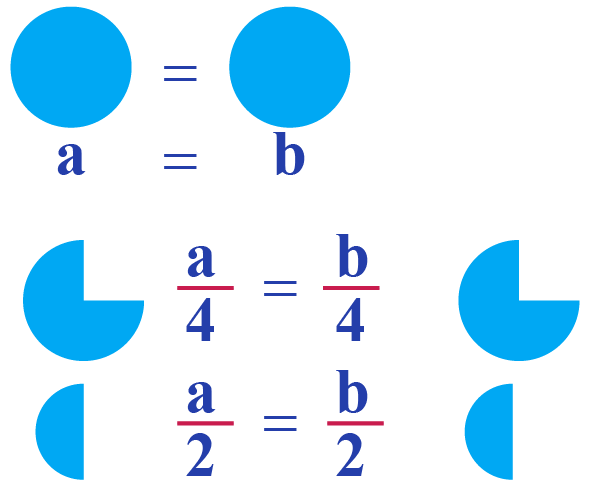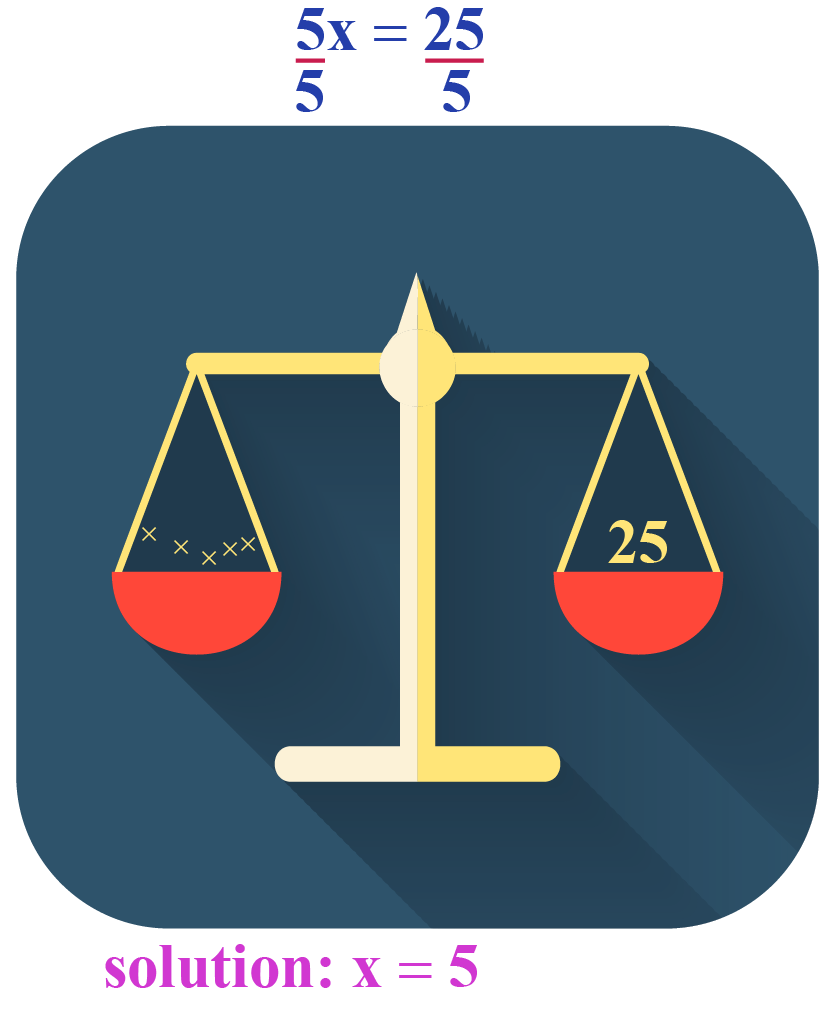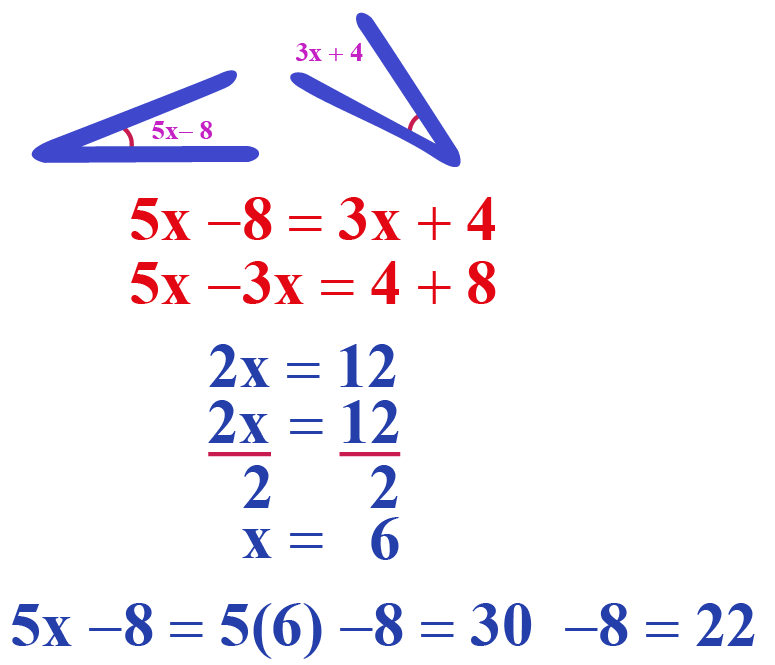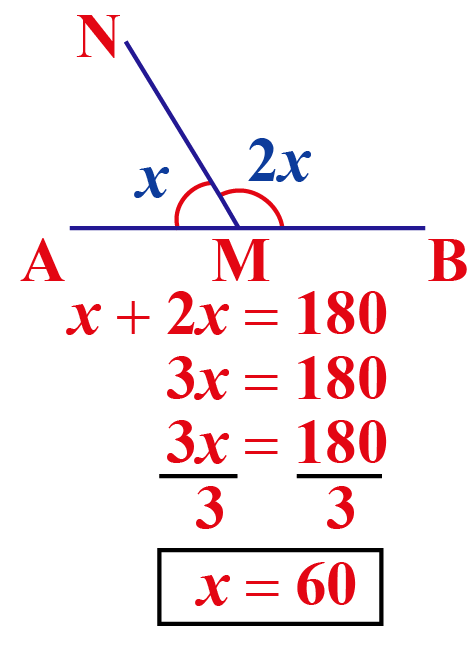# Division property of equality

Division property of equality

Jane has two cakes of equal sizes. She has 8 guests at the party. She decides to divide both the cakes evenly throughout. How will she be able to do this?Thus dividing both the cakes evenly to get the balance while using them is the division property of equality. Let's learn more about it in detail.

In this mini lesson let us learn about the division property of equality formula, division property of equality in geometry, division property of equality with fractions, division property of equality proof, division property of equality calculator and division property of equality angles.

## Lesson Plan

 1 What is Division Property of Equality? 2 Tips on Division Property of Equality 3 Important Notes on Division Property of Equality 4 Solved Examples on Division Property of Equality 5 Interactive Questions on Division Property of Equality

## What Is Division Property of Equality?

If $$a$$, $$b$$, $$c$$ are the real numbers, such that, then the division property of equality formula is given by:

 If $$a = b$$ and $$c \neq 0$$, then $$\dfrac{a}{c} = \dfrac{b}{c}$$

Consider the equation $$18 = 18$$

Divide both sides by 2

\begin{align}\dfrac{18}{2} &= \dfrac{18}{2}\\ 9 &= 9\\\text{LHS} &= \text{RHS}\end{align}

The equation still remains balanced.

### Definition

Division property states that when we divide one side of an equation by a number, we should divide the other side of the equation by the same number so that the equation remains balanced.

### Division Property of Equality Proof

The following picture illustrates the division property of equality in Algebra in solving linear equations.

If $$5x = 25$$, then $$x = 5$$ on dividing by 5 on both sides.

Thus, we apply the division property of equality formula here to solve for $$x$$You can verify if $$x = 5$$ is the solution of the given equation by substituting $$x = 5$$ in the equation.

\begin{align}\text{LHS} &=5 x\\ &= 5\times x\\ &= 5\times 5\\ &= 25\\ &= \text{RHS}\end{align}

Thus we obtain the division property of equality proof.

## Formula of Division Property of Equality

The formula of division property of equality is given as:

 If $$a = b$$ and $$c = d$$, then $$\dfrac{a}{c}= \dfrac{b}{d}$$

Example

1. To find the value of the unknown variable, we use this division property along with the other properties of equality.

Consider solving this linear equation, $$3x - 2 = 7$$

\begin{align*}3x - 2 + 2 &= 7 + 2 \text{ (addition property)}\\\\ 3x &= 9\\\\\dfrac{3x}{3} &= \dfrac{9}{3}\,\text{(division proerty})\\\\x &= 3\end{align*}

2. Consider equation $$2x = 1$$

To solve for $$x$$, divide both sides by 2.

\begin{align}\dfrac{2x}{2} &= \dfrac{1}{2}\,\text{(division property)}\\\\ x &= \dfrac{1}{2}\end{align}

### Division Property of Equality Calculator

In the following simulation, enter the equation, try to solve the equations and check your answers with the step-by-step procedure in solving the equation.Tips and Tricks
• When the equations to be solved are in the form of multiplication, we need to use the division property of equality. $$10 x = 100$$, here is the hint for us to use the division property. Divide both sides by 10.

### Division Property of Equality in Geometry

Congruent angles have equal measures.

1. Division Property of Equality in Geometry is used along with the properties of congruence.

Given are two congruent angles. We need to find the measures of the angles.

$$5x - 8$$ and $$3x + 4$$ are the two congruent angles.

$$\therefore 5x-8 = 3x +4$$

We need to solve for $$x$$ and then the angles by using the division property of equality angles.Thus we find that both the angles are equal to $$22^\circ$$

2. Division Property of Equality Angles is used to find the unknown angles.$$\angle AMN + \angle BMN = 180^\circ$$ $$\because$$ the angles form a linear pair and are supplementary.

\begin{align}x + 2x &= 180\\\\3x &= 180 (\text{division property)}\\\\ x &= 60\end{align}

### Division Property of Equality with Fractions

\begin{align}10\text{v} &= 2\\\\\dfrac{10 \text{ v}}{10} &= \dfrac{2}{10}\text{(divide both sides by 10)}\\\\\text{v} &= \dfrac{1}{5}\end{align}

You can verify if $$\text{ v} = \dfrac{1}{5}$$ by substituting in the equation.

\begin{align}\text{LHS} &= 10 \text{v}\\\\ &= 10\times \dfrac{1}{5}\\ &= 2\\\\ &=\text{RHS} \end{align}

Thus verified.

## What Are the 8 Properties of Equality?

The following are the 8 properties of equality:

Property Application
Substitution If $$a = b$$, then "b" can replace "a" in any expression.
Addition If $$a = b$$, then $$a + c = b + c$$
Subtraction If $$a = b$$, then $$a - c = b - c$$
Multiplication If $$a = b$$, then $$ac = bc$$
Division If $$a = b$$and  $$c \neq 0$$, then $$\dfrac{a}{c}= \dfrac{b}{c}$$
Symmetric if $$a= b$$, then $$b = a$$
Reflex $$a = a$$ i.e. the number is equal to itself
Transitive If $$a = b$$ and $$b = c$$ , then $$a = c$$
More Important Topics
More Important Topics